## Solution to 1986 Problem 24

 There are no changing magnetic fields in the problem, so we can apply Kirchhoff's Law around the loop.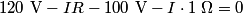\begin{align*}120 \mbox{ V} - I R - 100 \mbox{ V} - I \cdot 1 \;\Omega = 0\end{align*}where we have modeled the battery as an ideal voltage source of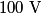$100 \mbox{ V}$ in series with a resistor of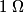$1 \;\Omega$. We are given that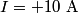$I = +10 \mbox{ A}$ (the problem statement does not explicitly tell us in which direction the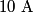$10 \mbox{ A}$ current is flowing, but if the current is assumed to flow in counterclockwise direction, then the resistance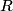$R$ turns out to be negative, which is absurd) and from this we can find the value of$R$: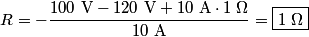\begin{align*}R = -\frac{100 \mbox{ V} - 120 \mbox{ V} + 10 \mbox{ A} \cdot 1 \;\Omega}{10 \mbox{ A}} = \boxed{1 \;\Omega}\en...Therefore, answer (C) is correct.